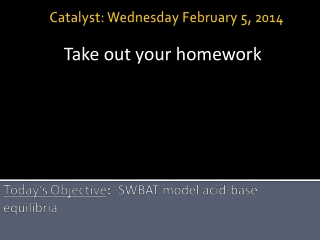DownloadDownload PresentationToday’s Objective : - SWBAT model acid-base equilibria

# Today’s Objective : - SWBAT model acid-base equilibria

Download Presentation## Today’s Objective : - SWBAT model acid-base equilibria

- - - - - - - - - - - - - - - - - - - - - - - - - - - E N D - - - - - - - - - - - - - - - - - - - - - - - - - - -
##### Presentation Transcript

1. Catalyst: Wednesday February 5, 2014 Take out your homework Today’s Objective: -SWBAT model acid-base equilibria

2. Review The pH of a 0.150 M solution of a weak acid is 4.10. Calculate the Ka value of the acid.

3. Announcements • Saturday School: 10-11ish • Monday: Cold Call Quiz

4. Photo of the day

5. Using ICE Tables How do we know when to use an ICE table to determine pH? Consider the following two problems • Determine the pH of a solution of 0.050 M HCl • Determine the pH of a solution of 0.080 M HF

6. Modeling Give each group a different problem Consider a solution of Weak Acid HA D H+ + A- • Determine the pH of the solution if [HA] = ? M • What species is present in the greatest concentration? • On your whiteboard, model the relative concentrations of each species HNO2 Ka = 4.0 x 10-4 HCOOH Ka= 1.77 x 10-4 HF Ka = 7.2 x 10-4

7. Conclusion • What can we conclude about the relative concentrations of each species of a weak acid at equilibrium? • What is present in greatest quantity? • What is present in the lowest quantity?

8. Discussion Question • Consider the following two solutions • HNO2: pH = 3.5 • HCl: pH = 3.5 Which solution has the greater concentration of hydronium ion in solution?

9. Lecture • Kw = Ka * Kb

10. Table Talk • Calculate the Ka for the ammonium ion (Kb = 1.8 x 10-5)

11. Table Talk • Consider the following two acids and their ionization constants: HCOOH Ka = 1.7 x 10-4 HCN Ka = 4.9 x 10-10 Which conjugate base (HCOO- or CN-) is stronger? Explain.

12. Lecture • Polyprotic acids: Acid that can donate more than one H+ • Calculations of Ka are more complicated because you need to take into account all potential ionizations • We’ll focus just on monoprotic acids • Acid Strength based on structure • Increasing size and EN leads to an increase in acid strength • Essentially, if molecule can ionize easier, it is a stronger acid • Increasing size and EN weakens bond

13. Lecture